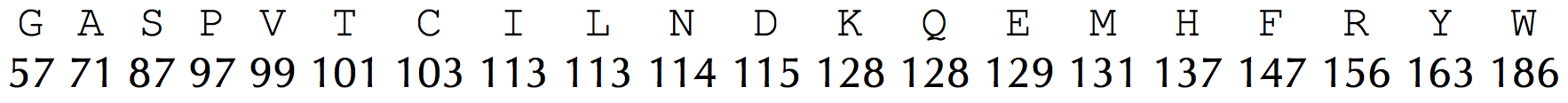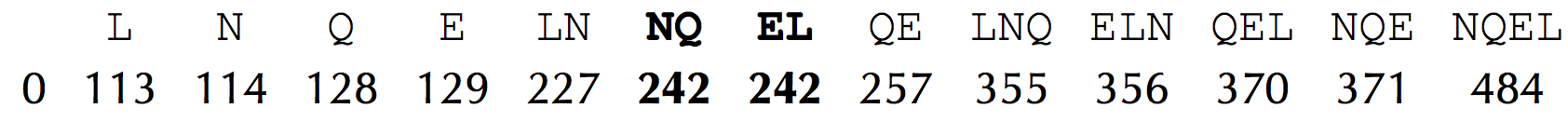# Generate the Theoretical Spectrum of a Cyclic Peptide solved by 744

July 29, 2015, 12:29 a.m. by Rosalind TeamFigure 1. Integer mass table for the 20 standard amino acids.Figure 2. Theoretical spectrum of NQEL, with NQ and EL highlighted.

The workhorse of peptide sequencing is the mass spectrometer, an expensive molecular scale that shatters molecules into pieces and then weighs the resulting fragments. The mass spectrometer measures the mass of a molecule in daltons (Da); 1 Da is approximately equal to the mass of a single nuclear particle (i.e., a proton or neutron).

We will approximate the mass of a molecule by simply adding the number of protons and neutrons found in the molecule’s constituent atoms, which yields the molecule’s integer mass. For example, the amino acid "Gly", which has chemical formula C2H3ON, has an integer mass of 57, since 2·12 + 3·1 + 1·16 + 1·14 = 57. Yet 1 Da is not exactly equal to the mass of a proton/neutron, and we may need to account for different naturally occurring isotopes of each atom when weighing a molecule. As a result, amino acids typically have non-integer masses (e.g., "Gly" has total mass equal to approximately 57.02 Da); for simplicity, however, we will work with the integer mass table given in Figure 1.

The theoretical spectrum of a cyclic peptide Peptide, denoted Cyclospectrum(Peptide), is the collection of all of the masses of its subpeptides, in addition to the mass 0 and the mass of the entire peptide. We will assume that the theoretical spectrum can contain duplicate elements, as is the case for "NQEL" (shown in Figure 2), where "NQ" and "EL" have the same mass.

## Generating Theoretical Spectrum Problem

Generate the theoretical spectrum of a cyclic peptide.

Given: An amino acid string Peptide.

Return: Cyclospectrum(Peptide).

## Sample Dataset

LEQN


## Sample Output

0 113 114 128 129 227 242 242 257 355 356 370 371 484